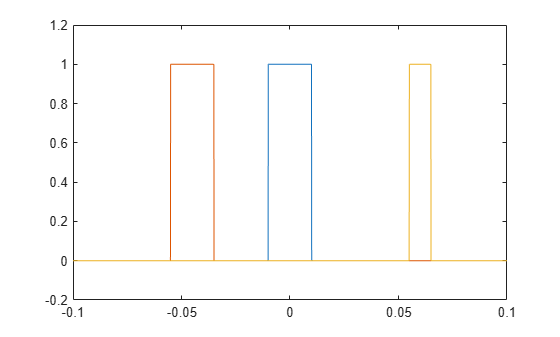# rectpuls

Sampled aperiodic rectangle

## Syntax

``y = rectpuls(t)``
``y = rectpuls(t,w)``

## Description

````y = rectpuls(t)` returns a continuous, aperiodic, unit-height rectangular pulse at the sample times indicated in array `t`, centered about `t` = `0`.```

example

````y = rectpuls(t,w)` generates a rectangle of width `w`.```

## Examples

collapse all

Generate 200 ms of a rectangular pulse with a sample rate of 10 kHz and a width of 20 ms.

```fs = 10e3; t = -0.1:1/fs:0.1; w = 20e-3; x = rectpuls(t,w);```

Generate two copies of the same pulse:

• One displaced 45 ms into the past.

```tpast = -45e-3; xpast = rectpuls(t-tpast,w);```
• One displaced 60 ms into the future and half as wide.

```tfutr = 60e-3; xfutr = rectpuls(t-tfutr,w/2);```

Plot the original pulse and the two copies on the same axes.

```plot(t,x,t,xpast,t,xfutr) ylim([-0.2 1.2])```## Input Arguments

collapse all

Sample times of unit rectangular pulse, specified as a vector.

Data Types: `single` | `double`

Rectangle width, specified as a positive number.

## Output Arguments

collapse all

Rectangular pulse of unit amplitude, returned as a vector.

Note

The interval of nonzero amplitude is defined to be open on the right, that is, `rectpuls(-0.5)` = `1` while `rectpuls(0.5)` = `0`.

## Tips

`rectpuls` can be used in conjunction with the pulse train generating function `pulstran`.

## Version History

Introduced before R2006a